# The figure shows three arrangements of a block attached to identical springs that are in their...

The figure shows three arrangements of a block attached to identical springs that are in their relaxed state when the block is centered as shown. Rank the arrangements according to the magnitude of the net force on the block, largest first, when the block is displaced by distance d (a) to the right and (b) to the left. Rank the arrangements according to the work done on the block by the spring forces, greatest first, when the block is displaced by d (c) to the right and (d) to the left.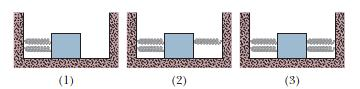JE (1) (2) (3)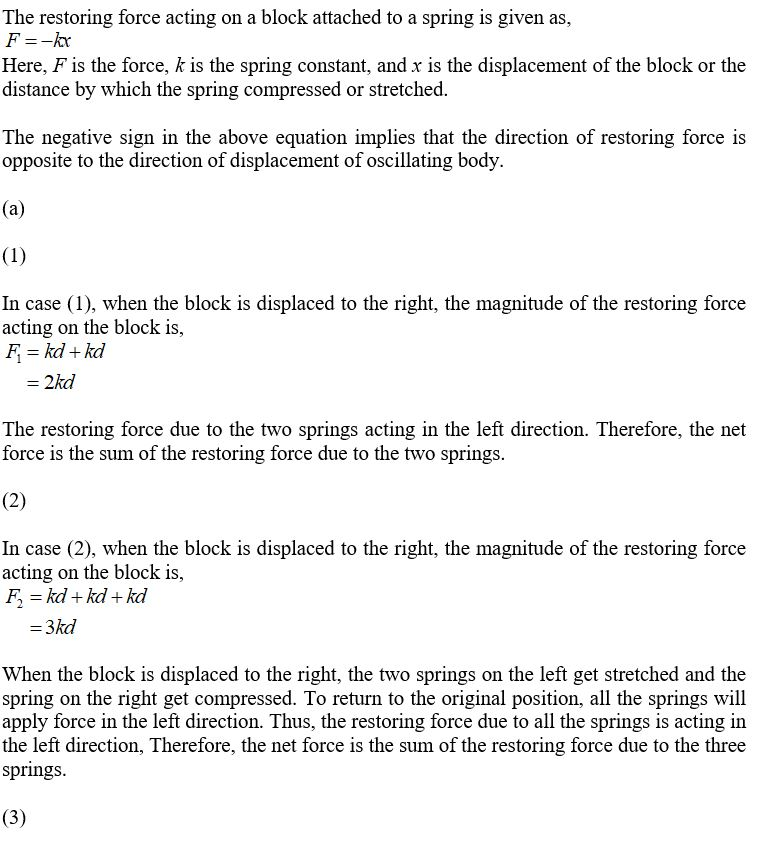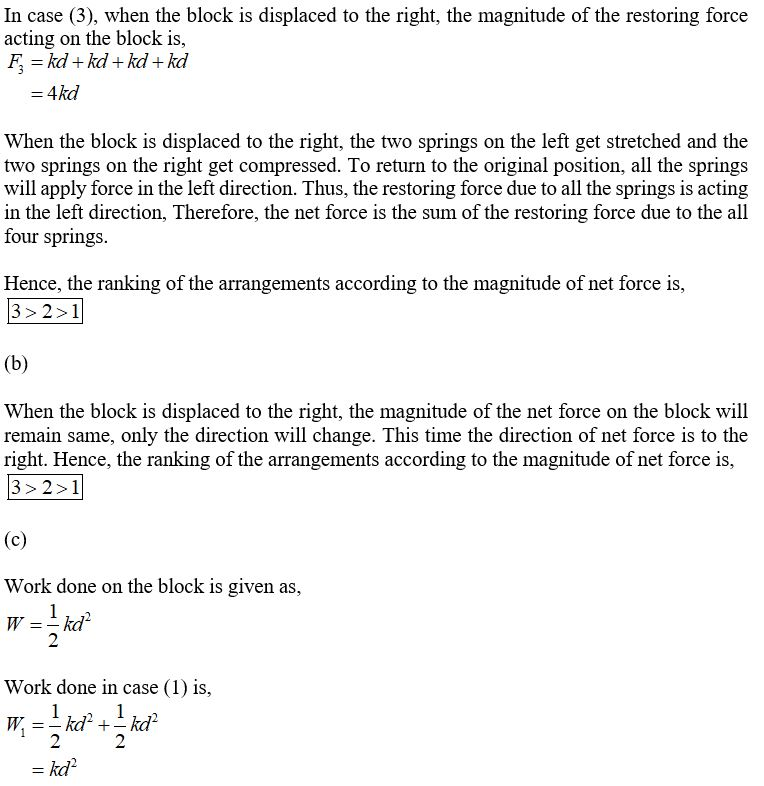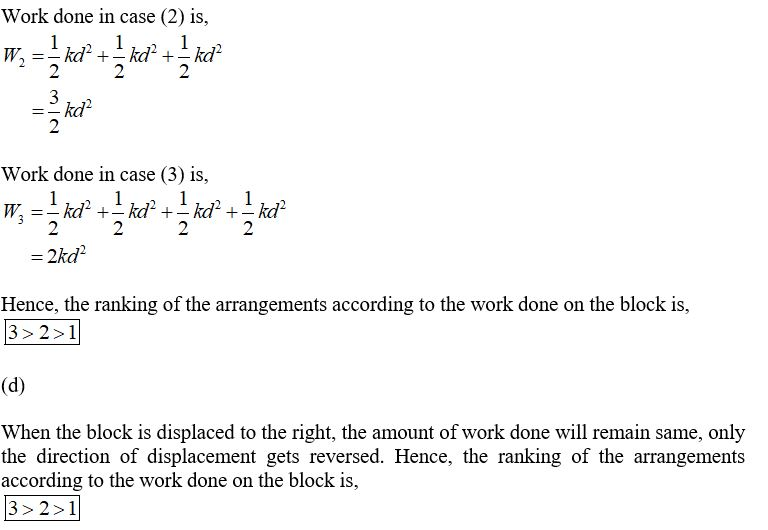#### Earn Coin

Coins can be redeemed for fabulous gifts.

Similar Homework Help Questions
• ### A block with mass m = 5.7 kg is attached to two springs with spring constants...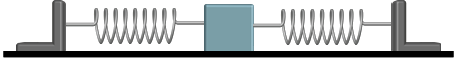A block with mass m = 5.7 kg is attached to two springs with spring constants kleft = 36 N/m and kright = 53 N/m. The block is pulled a distance x = 0.23 m to the left of its equilibrium position and released from rest. 1) What is the magnitude of the net force on the block (the moment it is released)?  2) What is the effective spring constant of the two springs?  3) What is the period of oscillation of...

• ### 1) The drawing shows three identical springs hanging from the ceiling. Nothing is attached to the...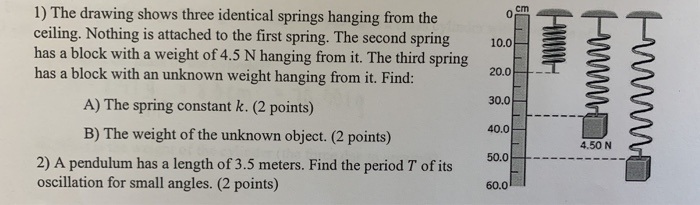1) The drawing shows three identical springs hanging from the ceiling. Nothing is attached to the first spring. The second spring has a block with a weight of 4.5 N hanging from it. The third spring has a block with an unknown weight hanging from it. Find: A) The spring constant k. (2 points) B) The weight of the unknown object. (2 points) 2) A pendulum has a length of 3.5 meters. Find the period T of its oscillation for...

• ### why is this the answer? The figure shows four arrangements in which long parallel wires carry...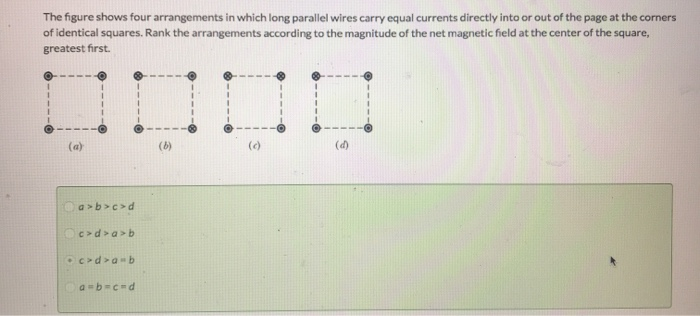why is this the answer? The figure shows four arrangements in which long parallel wires carry equal currents directly into or out of the page at the corners of identical squares. Rank the arrangements according to the magnitude of the net magnetic field at the center of the square, greatest first. a>b>c>d C> > > • coda-b a = b = c+d

• ### A block with mass m = 6.2 kg is attached to two springs with spring constants...A block with mass m = 6.2 kg is attached to two springs with spring constants kleft = 31 N/m and kright = 49 N/m. The block is pulled a distance x = 0.2 m to the left of its equilibrium position and released from rest. 1) What is the magnitude of the net force on the block (the moment it is released)? N 2) What is the effective spring constant of the two springs? N/m 3) What is the...

• ### The diagram shows three arrangements of circular loops, centered on vertical axes and carrying identical currents...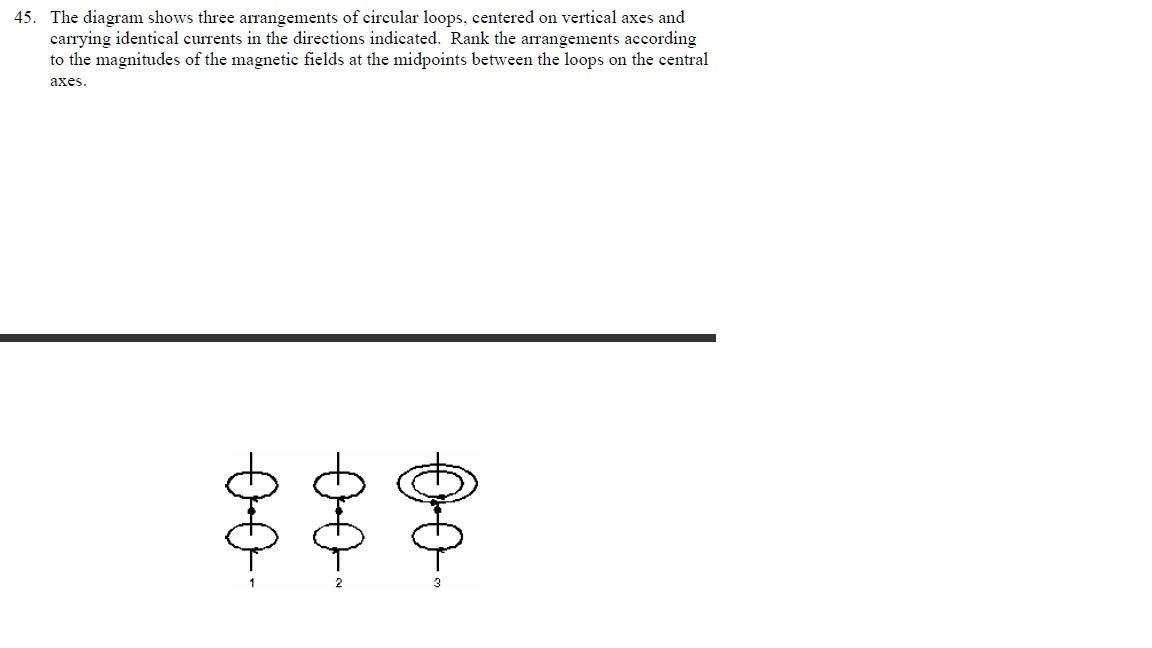The diagram shows three arrangements of circular loops, centered on vertical axes and carrying identical currents in the directions indicated. Rank the arrangements according to the magnitudes of the magnetic fields at the midpoints between the loops on the central axes.

• ### Simple Harmonic Motion Vidbook Pro SIMPLE HARMONIC MOTION Name Mech HW-79 Case A eum 1. Consider...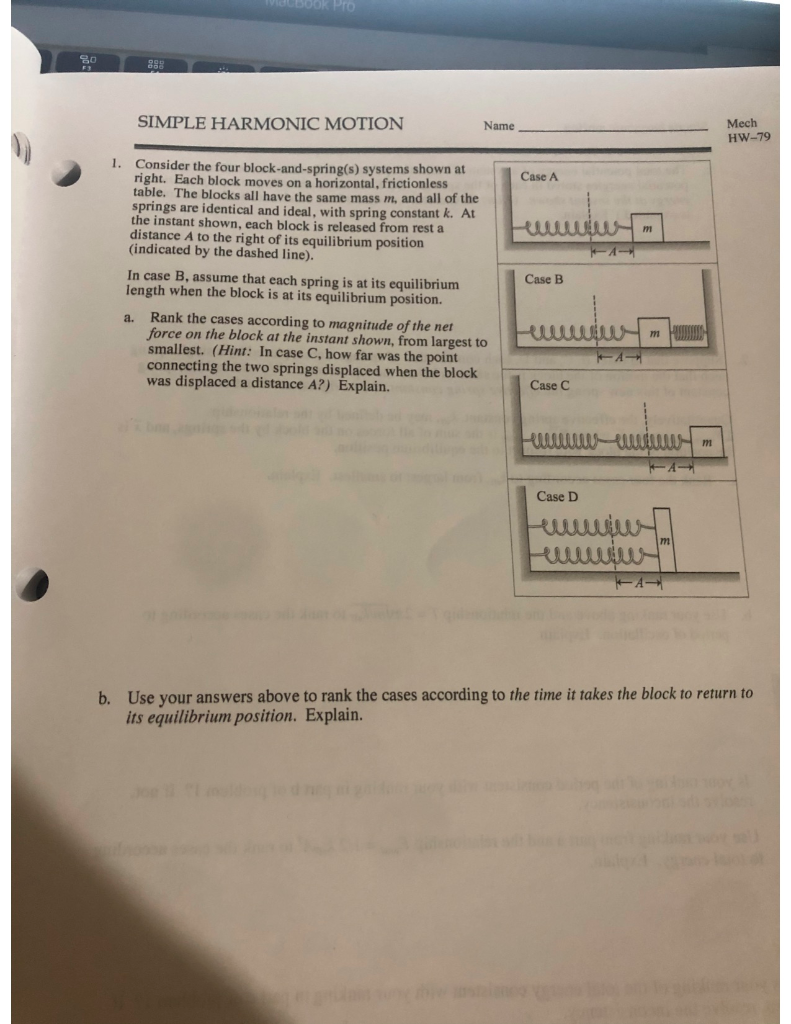Simple Harmonic Motion Vidbook Pro SIMPLE HARMONIC MOTION Name Mech HW-79 Case A eum 1. Consider the four block-and-spring(s) systems shown at right. Each block moves on a horizontal, frictionless table. The blocks all have the same mass m, and all of the springs are identical and ideal, with spring constant k. At the instant shown, each block is released from rest a distance A to the right of its equilibrium position (indicated by the dashed line). In case B,...

• ### A block with mass m = 7.4 kg is attached to two springs with spring constants...A block with mass m = 7.4 kg is attached to two springs with spring constants kleft = 31 N/m and kright = 53 N/m. The block is pulled a distance x = 0.27 m to the left of its equilibrium position and released from rest. 7) Where is the block located, relative to equilibrium, at a time 1.04 s after it is released? (if the block is left of equilibrium give the answer as a negative value; if the...

• ### www A block with mass m = 5.8 kg is attached to two springs with spring constants kleft = 37 N/m and Kright = 55 N/m. T...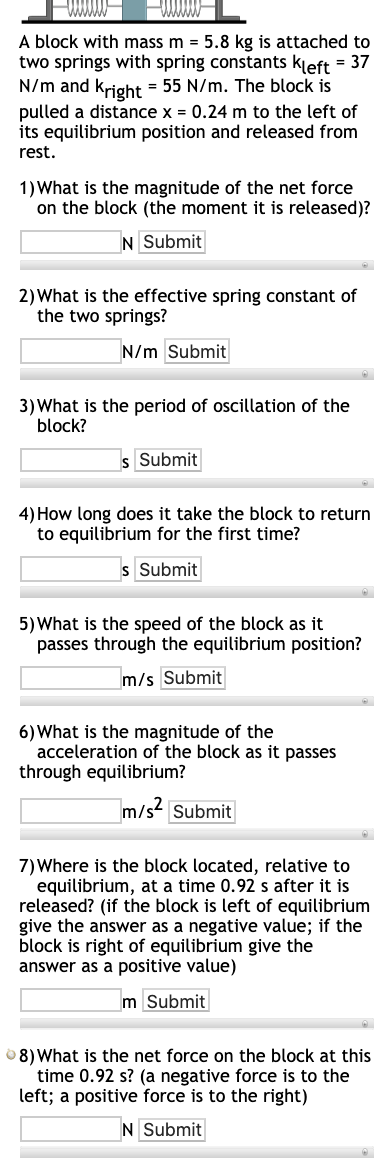www A block with mass m = 5.8 kg is attached to two springs with spring constants kleft = 37 N/m and Kright = 55 N/m. The block is pulled a distance x = 0.24 m to the left of its equilibrium position and released from rest. 1) What is the magnitude of the net force on the block (the moment it is released)? N Submit 2) What is the effective spring constant of the two springs? N/m Submit 3)...

• ### A block with mass m = 7 kg is attached to two springs with spring constants...

A block with mass m = 7 kg is attached to two springs with spring constants kleft = 37 N/m and kright = 48 N/m. The block is pulled a distance x = 0.21 m to the left of its equilibrium position and released from rest. 6)What is the magnitude of the acceleration of the block as it passes through equilibrium? 7)Where is the block located, relative to equilibrium, at a time 1.23 s after it is released? (if the...

• ### Q1 - Two identical blocks are attached to identical springs. The oscillation amplitude of Block A...

Q1 - Two identical blocks are attached to identical springs. The oscillation amplitude of Block A is 10cm; and the amplitude of Block B is 5 cm. Which block has a greater oscillation period? A- Block A B- Block B C- The periods are the same for both Blocks. Q2 - For an object in oscillation, which of the following is defined to be the maximum displacement from the equilibrium position. A- Period B- Frequency C- Angular frequency D- Amplitude...